Electron. J. Differential Equations, Vol. 2020 (2020), No. 08, pp. 1-26.

### Characterization of mean value harmonic functions on norm induced metric measure spaces with weighted Lebesgue measure Antoni Kijowski

Abstract:
We study the mean-value harmonic functions on open subsets of~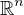equipped with weighted Lebesgue measures and norm induced metrics. Our main result is a necessary condition stating that all such functions solve a certain homogeneous system of elliptic PDEs. Moreover, a converse result is established in case of analytic weights. Assuming the Sobolev regularity of the weight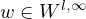we show that strongly harmonic functions are also in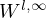and that they are analytic, whenever the weight is analytic.
The analysis is illustrated by finding all mean-value harmonic functions in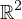for the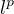-distance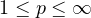. The essential outcome is a certain discontinuity with respect to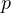, i.e. that for all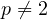there are only finitely many linearly independent mean-value harmonic functions, while for p=2 there are infinitely many of them. We conclude with the remarkable observation that strongly harmonic functions in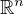possess the mean value property with respect to infinitely many weight functions obtained from a given weight.

Submitted March 21, 2019. Published January 14, 2020.
Math Subject Classifications: 31C05, 35J99, 30L99.
Key Words: Harmonic function; mean value property; metric measure space; Minkowski functional; norm induced metric; Pizzetti formula; weighted Lebesgue measure.

Show me the PDF file (427 KB), TEX file for this article.Antoni Kijowski Institute of Mathematics Polish Academy of Sciences, ul. Sniadeckich 8 00-656 Warsaw, Poland email: akijowski@impan.pl

Return to the EJDE web page